# Excel Functions List

Excel let’s you perform any operations using the Excel functions and their formulas. These functions can have the arguments input as cell references.

#### DATE & TIME

 FUNCTIONS DESCRIPTION SYNTAX DATE Returns the date in excel date-time code =DATE(year, month, day) DATEVALUE Converts a date in the form of text to a number that represents date in excel date-time code =DATEVALUE(date_text) DAY Returns the day of the month, a number within range 1-31 =DAY(serial_number) HOUR Returns the hour, a number within range 0-23 =HOUR(serial_number) MINUTE Returns the minute, a number within range 0-59 =MINUTE(serial_number) MONTH Returns the month, a number within range 1-12 =MONTH(serial_number) NOW Returns the current date and time =NOW() SECOND Returns the Second, a number within range 0- 59 =SECOND(serial_number) TIME Returns the number of a particular time in excel time format =TIME(hour,minute,second) TIMEVALUE Converts a time in the form of text to a serial number =TIMEVALUE(time_text) TODAY Returns the current date in excel date format =TODAY() DATEDIF Returns the period between two dates =DATEDIF (start_date, end_date, unit) DAYS Returns the days between the two dates =DAYS (end_date, start_date) DAYS360 Calculates the number of days between two dates based on a 360-day year(twelve 30-days month =DAYS360(start_date,end_date,method) EDATE Returns the serial number of the date that is the indicated number of months before or after the start date =EDATE(start_date,months) EOMONTH Returns the serial number of the last day of the month before or after a specified number of months =EOMONTH(start_date,months) NETWORDAYS Returns the elapsed days between two dates(excluding Saturday & Sunday) =NETWORKDAYS(start_date,end_date,[holidays]) WEEKDAY Returns a number(1-7) identifying the day of the week of a date =WEEKDAY(serial_number,[return_type]) WEEKNUM Returns the week number in the year =WEEKNUM(serial_number,[return_type]) ISOWEEKNUM Returns the ISO week number for a given date =ISOWEEKNUM(date WORKDAY Returns the serial number of the date before or after a specified number of workdays =WORKDAY(start_date, days, [holidays]) YEAR Returns the year of a date =YEAR(serial_number) YEARFRAC Returns the year fraction representing the number of whole days between start_date and end_date =YEARFRAC(start_date,end_date,basis)

#### LOGIC FUNCTIONS

 FUNCTIONS DESCRIPTION SYNTAX AND Checks all the logic arguments and returns True if all are True =AND(logical1,logical2,…) OR Checks all the logic arguments and returns False if all are False =OR(logical1,logical2,…) XOR Returns a logical “exclusive OR” of all arguments. =XOR(logical1,logical2,…) FALSE Returns the logical value FALSE =FALSE() TRUE Returns the logical value TRUE =TRUE() NOT Returns the opposite of the logic of its argument =NOT(logical) IF Checks the logic_test and returns one value if True and another value if False =IF(logical_test, [value_if_true], [value_if_false]) IFERROR Checks the logic_test and returns value if any error =IFERROR(value, value_if_error) IFNA Checks the logic_test and returns value if only #NA error occurs =IFNA(value, value_if_error) IFS Checks the multiple logic_tests. Same as using nested IF function =IFS (test1, value1, [test2, value2], …) ISBLANK Checks the cell and returns True if blank =ISBLANK(value) ISERROR Returns TRUE if the value is any error except #N/A else False =ISERR(value) ISERR Returns TRUE if the value is any error except #N/A else False =ISERR(value) ISEVEN Returns TRUE if the number is even =ISEVEN(number) ISLOGICAL Check if the value is a logical value =ISLOGICAL(value) ISFORMULA Check if the cell reference is a formula =ISFORMULA(reference) ISNA Checks if the value is the #N/A error =ISNA(value) ISNONTEXT Checks if the value is non-text =ISNONTEXT(value) ISNUMBER Checks of the value is a number =ISNUMBER(value) ISODD Returns TRUE if the number is odd =ISODD(number) ISREF Returns TRUE if the value is a reference =ISREF(value) ISTEXT Checks if the value is text =ISTEXT(value) N Converts non-number value to a number =N(value) NA Returns a #NA error =NA()

#### INFO FUNCTIONS

 FUNCTIONS DESCRIPTION SYNTAX CELL Returns information about the formatting, location, or contents of the first cell =CELL(info_type, [reference]) INFO Returns information about the current operating environment =INFO(type_text) SHEET Returns the sheet number of the referenced sheet =SHEET([value]) SHEETS Returns the number of sheets in a reference =SHEETS([reference]) T Checks the value and returns true if text =T(value) TYPE Returns a number ( 1, 2, 4, 16, 64), number representing each data type =TYPE(value)

#### MATH FUNCTIONS

 FUNCTIONS DESCRIPTION SYNTAX ABS Just converts all negative numbers to positive =ABS(number) AGGREGATE Returns an aggregate in a list or database =AGGREGATE(function_num,options,array,k) ARABIC Converts a Roman numeral to Arabic =ARABIC(text) ASIN Returns the arcsine of a number =ASIN(number) CEILING Rounds a number to the nearest integer or to the nearest multiple of significance =CEILING(number,significance) DECIMAL Converts a text representation of a number in a given base into a decimal number =DECIMAL(number, radix) DEGREES Converts all radians to degrees =DEGREES(angle) EVEN Rounds a number up to the nearest even integer =EVEN(number) EXP Returns e (Value of e = 2.71..) raised to the power of a given number =EXP(number) FACT Returns the factorial of a number =FACT(number) FACTDOUBLE Returns the double factorial of a number =FACTDOUBLE(number) FLOOR Rounds a number down nearest multiple of significance =FLOOR(number,significance) GCD Returns the greatest common divisor =GCD(number1,number2,…) INT Rounds a number down to the nearest integer =INT(number) LCM Returns the least common multiple =LCM(number1,number2,…) LN Returns the natural logarithm of a number =LN(number) LOG Returns the logarithm of a number to a specified base =LOG(number,base) LOG10 Returns the base-10 logarithm of a number =LOG10(number) MDETERM Returns the matrix determinant of an array =MDETERM(array) MINVERSE Returns the matrix inverse of an array =MINVERSE(array) MOD Returns the most occuring value in a data set of values =MOD(number, divisor) MROUND Returns a number rounded to the desired multiple =MROUND(number,multiple) MUNIT Returns the unit matrix for the specified dimensions =MUNIT(dimension) ODD Rounds a number up to the nearest odd integer =ODD(number) PI Returns the value of pi, 3.14159….upto to 15 digits =PI() POWER Returns the result of a number raised to a power =POWER(number,power) PRODUCT Multiplies its arguments, text & logic statements ignored =PRODUCT(number 1, number 2,…) QUOTIENT Returns the integer portion of a division =QUOTIENT(numerator,denominator) RADIANS Converts degrees to radians =RADIANS(angle) RAND Returns a random number range 0 number < 1 =RAND() RANDBETWEEN Returns a random number between the two numbers =RANDBETWEEN(bottom,top) ROMAN Converts an arabic numeral to roman, as text =ROMAN(number,form) ROUND Rounds a number to a specified number of digits =ROUND(number,num_digits) ROUNDDOWN Rounds a number down, toward zero =ROUNDDOWN(number,num_digits) ROUNDUP Rounds a number up, away from zero =ROUNDUP(number,num_digits) SIGN Checks the number, returns 1 if positive, o if negative and -1 if negative =SIGN(number) SIN Returns the sine of the given angle =SIN(number) SINH Returns the hyperbolic sine of a number =SINH(number) COS Returns the cosine of a number =COS(number) COSH Returns the hyperbolic cosine of a number =COSH(number) TAN Returns the tangent of a number =TAN(number) TANH Returns the hyperbolic tangent of a number =TANH(number) SQRT Returns a square root of a number =SQRT(number) SUBTOTAL Returns a subtotal in a list or database =SUBTOTAL(function_num,ref1,…) SUM Adds all the numbers in a range of cells. =SUM(number1,number2,…) SUMIF Adds the cells specified by a given condition or criteria =SUMIF(range,criteria,[sum_range]) SUMIFS Adds the cells specified by a given set of conditions or criteria =SUMIFS(sum_range,criteria_range,criteria,…) SUMPRODUCT Returns the sum of the products of corresponding ranges or arrays =SUMPRODUCT(array1,array2,[array3],…) TRUNC Truncates a number to an integer removing the decimal part of the number =TRUNC(number,num_digits) ACOS Returns the arccosine of a number =ACOS(number) ACOSH Returns the inverse hyperbolic cosine of a number =ACOSH(number) ASIN Returns the arcsine of a number =ASIN(number) ASINH Returns the inverse hyperbolic sine of a number =ASINH(number) ATAN Returns the arctangent of a number =ATAN(number) ATAN2 Returns the arctangent from x- and y-coordinates =ATAN2(x_num,y_num) ATANH Returns the inverse hyperbolic tangent of a number =ATANH(number) BAHTTEXT Converts a number to text, using the ß (baht) currency format =BAHTTEXT(number) CUBEKPIMEMBER Returns a key performance indicator (KPI) name, property, and measure, and displays the name and property in the cell. A KPI is a quantifiable measurement, such as monthly gross profit or quarterly employee turnover, used to monitor an organization’s performance. =CUBEKPIMEMBER(connection,kpi_name, kpi_property,caption) CUBEMEMBER Returns a member or tuple in a cube hierarchy. Use to validate that the member or tuple exists in the cube. =CUBEMEMBER(connection, member_expression,caption) CUBEMEMBERPROPERTY Returns the value of a member property in the cube. Use to validate that a member name exists within the cube and to return the specified property for this member. =CUBEMEMBERPROPERTY(connection, member_expression,property) CUBERANKEDMEMBER Returns the nth, or ranked, member in a set. Use to return one or more elements in a set, such as the top sales performer or top 10 students. =CUBERANKEDMEMBER(connection, set_expression,rank,caption) CUBESET Defines a calculated set of members or tuples by sending a set expression to the cube on the server, which creates the set, and then returns that set to Microsoft Office Excel. =CUBESET(connection,set_expression, caption,sort_order,sort_by) CUBESETCOUNT Returns the number of items in a set. =CUBESETCOUNT(set) CUBEVALUE Returns an aggregated value from a cube. =CUBEVALUE(connection, member_expression1,…)

#### DATABASE FUNCTIONS

 FUNCTIONS DESCRIPTION SYNTAX DAVERAGE Returns the average of the values in the range that match conditions you specify =DAVERAGE(database,field,criteria) DCOUNT Counts the cells containing numbers in database matching conditions you specify =DCOUNT(database,field,criteria) DMAX Returns the maximum value from database matching conditions you specify =DMAX(database,field,criteria) DMIN Returns the minimum value from database matching conditions you specify =DMIN(database,field,criteria) DPRODUCT Multiplies the values in a particular field of records that match the criteria in a database =DPRODUCT(database,field,criteria) DSTDEV Estimates the standard deviation based on a sample of selected database entries =DSTDEV(database,field,criteria) DSTDEVP Calculates the standard deviation based on the entire population of selected database entries =DSTDEVP(database,field,criteria) DVAR Estimates variance based on a sample from selected database entries =DVAR(database,field,criteria) DVARP Calculates variance based on the entire population of selected database entries =DVARP(database,field,criteria) DGET Extracts from a database a single record that matches the specified criteria =DGET(database,field,criteria) DSUM Adds the numbers in the field column of records in the database that match the criteria you specify =DSUM(database,field,criteria) DCOUNTA Returns the number of nonblank cells in the database that matches a specific condition =DCOUNTA(database,field,criteria)

#### ENGINEERING FUNCTIONS

 FUNCTIONS DESCRIPTION SYNTAX BIN2DEC Converts a binary number to decimal =BIN2DEC(number) BIN2HEX Converts a binary number to hexadecimal =BIN2HEX(number,places) BIN2OCT Converts a binary number to octal =BIN2OCT(number,places) CONVERT Converts a number from one measurement system to another measurement =CONVERT(number,from_unit,to_unit) COMPLEX Converts real and imaginary coefficients into a complex number =COMPLEX(real_num,i_num,suffix) DEC2BIN Converts a decimal number to binary =DEC2BIN(number,places) DEC2HEX Converts a decimal number to hexadecimal =DEC2HEX(number,places) DEC2OCT Converts a decimal number to octal =DEC2OCT(number,places) HEX2BIN Converts a hexadecimal number to binary =HEX2BIN(number,places) HEX2DEC Converts hexadecimal number to decimal =HEX2DEC(number) HEX2OCT Converts a hexadecimal number to octal =HEX2OCT(number,places) IMABS Returns the absolute value (modulus) of a complex number =IMABS(inumber) IMAGINARY Returns the imaginary coefficient of a complex number =IMAGINARY(inumber) IMARGUMENT Returns the argument theta, an angle expressed in radians =IMARGUMENT(inumber) IMCONJUGATE Returns the complex conjugate of a complex number =IMCONJUGATE(inumber) IMCOS Returns the cosine of a complex number =IMCOS(inumber) IMDIV Returns the quotient of two complex numbers =IMDIV(inumber1,inumber2) IMEXP Returns the exponential of a complex number =IMEXP(inumber) IMLN Returns the natural logarithm of a complex number =IMLN(inumber) IMLOG10 Returns the base-10 logarithm of a complex number =IMLOG10(inumber) IMLOG2 Returns the base-2 logarithm of a complex number =IMLOG2(inumber) IMPOWER Returns a complex number raised to an integer power =IMPOWER(inumber,number) IMPRODUCT Returns the product of complex numbers =IMPRODUCT(inumber1,inumber2,…) IMREAL Returns the real coefficient of a complex number =IMREAL(inumber) IMSIN Returns the sine of a complex number =IMSIN(inumber) IMSQRT Returns the square root of a complex number =IMSQRT(inumber) IMSUB Returns the difference between two complex numbers =IMSUB(inumber1,inumber2) IMSUM Returns the sum of complex numbers =IMSUM(inumber1,inumber2,…) OCT2BIN Converts an octal number to binary =OCT2BIN(number,places) OCT2DEC Converts an octal number to decimal =OCT2DEC(number) OCT2HEX Converts an octal number to hexadecimal =OCT2HEX(number,places) BITAND Returns the bitwise ‘AND’ of two numbers =BITAND(number1, number2) BITOR Returns the bitwise ‘OR’ of two numbers =BITOR(number1, number2) BITXOR Returns the bitwise ‘Exclusive OR’ of two numbers =BITXOR(number1, number2) BITLSHIFT Returns a number shifted left by shift_amount bits =BITLSHIFT(number, shift amount) BITRSHIFT Returns a number shifted right by shift_amount bits =BITRSHIFT(number, shift amount) DELTA Checks the two values are equal or not =DELTA(number1, [number2])

#### FINANCIAL FUNCTIONS

 FUNCTIONS DESCRIPTION SYNTAX ACCRINT Returns the accrued interest for a security that pays periodic interest =ACCRINT(issue,first_interest, settlement,rate,par,frequency, basis,calc_method) ACCRINTM Returns the accrued interest for a security that pays interest at maturity =ACCRINTM(issue,settlement, rate,par,basis) AMORDEGRC Returns the prorated linear depreciation of an asset for each accounting period =AMORDEGRC(cost,date_purchased, first_period,salvage,period, rate,[basis]) AMORLINC Returns the prorated linear depreciation of an asset for each accounting period =AMORLINC(cost,date_purchased, first_period,salvage, period,rate,basis) COUPDAYBS Returns the number of days from the beginning of the coupon period to the settlement date =COUPDAYBS(settlement,maturity, frequency,basis) COUPDAYS Returns the number of days in the coupon period that contains the settlement date =COUPDAYS(settlement,maturity, frequency,basis) COUPDAYSNC Returns the number of days from the settlement date to the next coupon date =COUPDAYSNC(settlement,maturity, frequency,basis) COUPNCD Returns the next coupon date after the settlement date =COUPNCD(settlement,maturity, frequency,basis) COUPNUM Returns the number of coupons payable between the settlement date and maturity date =COUPNUM(settlement,maturity, frequency,basis) COUPPCD Returns the previous coupon date before the settlement date =COUPPCD(settlement,maturity, frequency,basis) CUMIPMT Returns the cumulative interest paid between two periods =CUMIPMT(rate,nper,pv, start_period,end_period,type) CUMPRINC Returns the cumulative principal paid on a loan between two periods =CUMPRINC(rate,nper,pv, start_period,end_period,type) DB Returns the depreciation of an asset for a specified period by using the fixed-declining balance method =DB(cost,salvage,life, period,month) DDB Returns the depreciation of an asset for a specified period by using the double-declining balance method or some other method that you specify =DDB(cost,salvage,life, period,factor) DISC Returns the discount rate for a security =DISC(settlement,maturity, pr,redemption,basis) DOLLARDE Converts a dollar price, expressed as a fraction, into a dollar price, expressed as a decimal number =DOLLARDE(fractional_dollar, fraction) DOLLARFR Converts a dollar price, expressed as a decimal number, into a dollar price, expressed as a fraction =DOLLARFR(decimal_dollar,fraction) DURATION Returns the annual duration of a security with periodic interest payments =DURATION(settlement,maturity, coupon,yld,frequency,basis) EFFECT Returns the effective annual interest rate =EFFECT(nominal_rate,npery) FV Returns the future value of an investment =FV(rate,nper,pmt,pv,type) IPMT Returns the interest payment for an investment for a given period for an investment =IPMT(rate,per,nper,pv,fv,type) FVSCHEDULE Returns the future value of an initial principal after applying a series of compound interest rates =FVSCHEDULE(principal,schedule) INTRATE Returns the interest rate for a fully invested security =INTRATE(settlement,maturity, investment,redemption,basis) ISPMT Calculates the interest paid during a specific period of an investment =ISPMT(rate,per,nper,pv) IRR Returns the internal rate of return for a series of cash flows =IRR(values,guess) MDURATION Returns the Macauley modified duration for a security with an assumed par value of \$100 =MDURATION(settlement,maturity, coupon,yld,frequency,basis) MIRR Returns the internal rate of return for a series of periodic cash flows, considering both debit and credit cash flow =MIRR(values,finance_rate,reinvest_rate) NOMINAL Returns the annual nominal interest rate =NOMINAL(effect_rate,npery) NPER Returns the number of periods for an investment at constant rate and fixed monthly amount =NPER(rate,pmt,pv,fv,type) NPV Returns the net present value of an investment based on a series of periodic cash flows and a discount rate and a series of future payments =NPV(rate,value1,value2,…) PV Returns the present value of an investment =PV(rate,nper,pmt,fv,type) RATE Returns the interest rate per period of an annuity =RATE(nper,pmt,pv,fv,type,guess) ODDFPRICE Returns the price per \$100 face value of a security with an odd first period =ODDFPRICE(settlement,maturity, issue,first_coupon,rate,yld, redemption,frequency,basis) ODDFYIELD Returns the yield of a security with an odd first period =ODDFYIELD(settlement,maturity, issue,first_coupon,rate,pr, redemption,frequency,basis) ODDLYIELD Returns the yield of a security with an odd last period =ODDLPRICE(settlement,maturity, last_interest,rate,pr, redemption,frequency,[basis]) ODDLPRICE Returns the price per \$100 face value of a security with an odd last period =ODDLYIELD(settlement,maturity, last_interest,rate,yield, redemption,frequency,[basis]) PMT Returns the periodic payment for an annuity =PMT(rate,nper,pv,fv,type) PPMT Returns the payment on the principal for an investment for a given period =PPMT(rate,per,nper,pv,fv,type) PRICE Returns the price per \$100 face value of a security that pays periodic interest =PRICE(settlement,maturity,rate, yld,redemption,frequency,basis) PRICEDISC Returns the price per \$100 face value of a discounted security =PRICEDISC(settlement,maturity, discount, redemption, [basis]) PRICEMAT Returns the price per \$100 face value of a security that pays interest at maturity =PRICEMAT(settlement,maturity, issue,rate,yld,basis) RECEIVED Returns the amount received at maturity for a fully invested security =RECEIVED(settlement,maturity, investment,discount,basis) RRI Returns an equivalent interest rate for the growth of an investment =RRI(nper, pv, fv) SLN Returns the straight-line depreciation of an asset for one period =SLN(cost,salvage,life) SYD Returns the sum-of-years’ digits depreciation of an asset for a specified period =SYD(cost,salvage,life,per) TBILLEQ Returns the bond-equivalent yield for a Treasury bill =TBILLEQ(settlement,maturity,discount) TBILLPRICE Returns the price per \$100 face value for a Treasury bill =TBILLPRICE(settlement,maturity,discount) TBILLYIELD Returns the yield for a Treasury bill =TBILLYIELD(settlement,maturity,pr) VDB Returns the depreciation of an asset for a specified or partial period by using a declining balance method =VDB(cost,salvage,life, start_period,end_period, factor,no_switch) XIRR Returns the internal rate of return for a schedule of cash flows that is not necessarily periodic =XIRR(values,dates,guess) XNPV Returns the net present value for a schedule of cash flows that is not necessarily periodic =XNPV(rate,values,dates) YIELD Returns the yield on a security that pays periodic interest =YIELD(settlement,maturity, rate,pr,redemption, frequency,basis) YIELDDISC Returns the annual yield for a discounted security; for example, a Treasury bill =YIELDDISC(settlement,maturity, pr,redemption,basis) YIELDMAT Returns the annual yield of a security that pays interest at maturity =YIELDMAT(settlement,maturity, issue,rate,pr,basis)

#### STATISTICS FUNCTIONS

 FUNCTIONS DESCRIPTION SYNTAX AVEDEV Returns the average of the absolute deviations of data points from their mean =AVEDEV(number1,number2,…) AVERAGE Returns the average(arithmetic mean) of its arguments =AVERAGE(number1,number2,…) AVERAGEIF Returns the average (arithmetic mean) of all the cells in a range that meet a given condition or criteria =AVERAGEIF(range,criteria,[average_range]) AVERAGEA Returns the average of its arguments, including numbers, text, and logical values =AVERAGEA(value1,value2,…) AVERAGEIFS Returns the average (arithmetic mean) of all cells that meet multiple criteria. =AVERAGEIFS(average_range, criteria_range,criteria,…) COMBIN Returns the number of combinations for a given number of values =COMBIN(number,number_chosen) COUNT Counts the number of cells in a range that contain numbers. =COUNT(value1,value2,…) COUNTA Count the number of non-empty cells in a range of cells. =COUNTA(value1,value2,…) COUNTBLANK Count the number of non-empty cells in a range of cells. =COUNTBLANK(range) COUNTIF Counts the number of cells within a range that meet the given condition =COUNTIF(range,criteria) COUNTIFS Counts the number of cells that meet a specified set of conditions or criteria =COUNTIFS(criteria_range,criteria,…) DEVSQ Returns the exponential distribution =EXPON.DIST(x,lambda,cumulative) FREQUENCY Returns a frequency distribution as a vertical array =FREQUENCY(data_array,bins_array) GEOMEAN Returns the geometric mean of the range of positive numeric values =GEOMEAN(number1,number2,…) HARMEAN Returns the harmonic mean =HARMEAN(number1,number2,…) LARGE Returns the k-th largest value in a data set =LARGE(array,k) MIN Returns the numerically smallest value, ignores logical values and text =MIN(number1,number2,…) MAX Returns the numerically largest value, ignores logical values and text =MAX(number1,number2,…) MAXA Returns the maximum value in a set of values, does not ignore text, and logical values =MAXA(value1,value2,…) MINA Returns the minimum value in a set of values, does not ignore text, and logical values =MINA(value1,value2,…) MEDIAN Returns the median of the set of given numbers =MEDIAN(number1,number2,…) MODE Returns the most occuring value in a data set of values =MODE(number1,number2,…) PERCENTILE Returns the k-th percentile of values in a range =PERCENTILE(array,k) PERCENTRANK Returns the percentage rank of a value in a data set =PERCENTRANK(array,x,significance) PERMUT Returns the number of permutations for a given number of objects =PERMUT(number,number_chosen) QUARTILE Returns the quartile of a data set =QUARTILE(array,quart) RANK Returns the rank of a number in a list of numbers =RANK(number,ref,order) SMALL Returns the k-th smallest value in a data set =SMALL(array,k) STANDARDIZE Returns a normalized value =STANDARDIZE(x,mean,standard_dev) STDEV Estimates standard deviation based on a sample =STDEV(number1,number2,…) STDEVA Estimates standard deviation based on a sample, including numbers, text, and logical values =STDEVA(value1,value2,…) STDEVP Calculates standard deviation based on the entire population =STDEVP(number1,number2,…) STDEVPA Calculates standard deviation based on the entire population, including numbers, text, and logical values =STDEVPA(value1,value2,…) TRIMMEAN Returns the mean of the interior of a data set =TRIMMEAN(array,percent) VAR Estimates variance based on a sample =VAR(number1,number2,…) VARA Estimates variance based on a sample, including numbers, text, and logical values =VARA(value1,value2,…) VARP Calculates variance based on the entire population =VARP(number1,number2,…) VARPA Calculates variance based on the entire population, including numbers, text, and logical values =VARPA(value1,value2,…)

#### LOOKUP & REFERENCE

 FUNCTIONS DESCRIPTION SYNTAX ADDRESS Returns a reference as text to a single cell in a worksheet =ADDRESS(row_num, column_num, [abs_num], [a1], [sheet_text]) AREAS Returns the number of areas in a reference =AREAS(reference) CHOOSE Chooses a value or action to perform from a list of values, based on an index number =CHOOSE(index_num,value1,value2,…) COLUMN Returns the column number of a reference =COLUMN([reference]) COLUMNS Returns the number of columns in an array or reference =COLUMNS(array) FORMULATEXT Returns a formula as a string =FORMULATEXT(reference) GETPIVOTDATA Extracts data stored in a pivotTable =GETPIVOTDATA(data_field,pivot_table,field,item,…) HLOOKUP Looks in the top row of an array and returns the value from the corresponding column =HLOOKUP(lookup_value,table_array,row_index_num,[range_lookup]) HYPERLINK Creates a shortcut or jump that opens a document from the link =HYPERLINK(link_location,friendly_name) INDEX Uses an index to choose a value from a reference or array =INDEX(array,row_num,[column_num]) INDIRECT Returns a reference indicated by a text value =INDIRECT(ref_text,a1) LOOKUP Looks up in the array and returns the value from the corresponding array =LOOKUP(lookup_value, array,[result vector]) MATCH Looks up for relative values in an array and returns the relative index of the match =MATCH(lookup_value,lookup_array,[match_type]) MMULT Returns the matrix product of two arrays =MMULT(array1,array2) OFFSET Returns a reference, traverses from a given reference =OFFSET(reference,rows,cols,height,width) ROW Returns the row number of a reference =ROW([reference]) ROWS Returns the number of rows in a reference or array =ROWS(array) TRANSPOSE Returns the transpose of an array (Rows to columns or vice versa) =TRANSPOSE(array) VLOOKUP Looks in the top column of an array and returns the value from the corresponding row =VLOOKUP(lookup_value,table_array,col_index_num,[range_lookup])

#### TEXT FUNCTIONS

 FUNCTIONS DESCRIPTION SYNTAX CHAR Returns the character specified by the code number used by your computer =CHAR(number) CLEAN Removes all non-printable characters from the text =CLEAN(text) CODE Returns a numeric code for the first character in the text string used by your computer =CODE(text) CONCATENATE Joins several text items into one text string. =CONCATENATE(text1,text2,…) DOLLAR Converts a number to text, using the dollar (\$) currency format =DOLLAR(number,decimals) EXACT Checks two text strings, if same returns True else False =EXACT(text1,text2) FIND Returns the starting position, where two strings match (case-sensitive) =FIND(find_text,within_text,start_num) LEFT Returns the specified number of characters from the start of the text string =LEFT(text,num_chars) LEN Returns the number of characters in a text string =LEN(text) LOWER Converts all the text to Lower Case =LOWER(text) MID Returns the specified characters from a given position of the text =MID(text,start_num,num_chars) PROPER Converts all the text to proper case. only First letter capital =PROPER(text) REPLACE Replaces part of a text string with a different text string =REPLACE(old_text,start_num,num_chars,new_text) RIGHT Returns the specified number of characters from the start of the Right string =RIGHT(text,num_chars) FIXED Formats a number as text with a specified number of decimals =FIXED(number,decimals,no_commas) TEXT Converts a value to text in a specific number format =TEXT(value,format_text) NUMBERVALUE Converts text to number in a locale-independent manner =NUMBERVALUE(text,[decimal_seperator],[group seperator]) REPT Repeats text a given number of times =REPT(text,number_times) SUBSTITUTE Replaces existing text with new text in a text string =SUBSTITUTE(text,old_text,new_text,instance_num) SEARCH Finds one text string within another string (not case-sensitive) =SEARCH(find_text,within_text,start_num) TRIM Removes all spaces from a text string except for single spaces between words =TRIM(text) UPPER Converts all the text to Upper Case =UPPER(text) UNICHAR Returns the unicode character of the number =UNICHAR(number) UNICODE Returns the number(code) corresponding to the first character of the text =UNICODE(text) VALUE Converts a text string to a number =VALUE(text)

#### EXTENSION (.) EXCEL FUNCTIONS AND FORMULAS

 FUNCTIONS DESCRIPTION SYNTAX NETWORKDAYS.INTL Returns the number of whole workdays between two dates using parameters to indicate which and how many days are weekend days =NETWORKDAYS.INTL( start_date,end_date, [weekend],[holidays]) WORKDAY.INTL Returns the serial number of the date before or after a specified number of workdays using parameters to indicate which and how many days are weekend days =WORKDAY.INTL( start_date,days,weekend, holidays) ERROR.TYPE Returns a number corresponding to an error type =ERROR.TYPE(error_val) CEILING.PRECISE Rounds a number the nearest integer or to the nearest multiple of significance. Regardless of the sign of the number, the number is rounded up. =CEILING.PRECISE( number,significance) FLOOR.PRECISE Rounds a number the nearest integer or to the nearest multiple of significance. Regardless of the sign of the number, the number is rounded up. =FLOOR.PRECISE( number,significance) ISO.CEILING Returns a number that is rounded up to the nearest integer or to the nearest multiple of significance =ISO.CEILING( number,significance) MODE.MULT Returns a vertical array of the most frequently occurring, or repetitive values in an array or range of data =MODE.MULT(number1, number2,…) MODE.SNGL Returns the most common value in a data set =MODE.SNGL(number1, number2,…) RANK.AVG Returns the rank of a number in a list of numbers =RANK.AVG(number, ref,order) RANK.EQ Returns the rank of a number in a list of numbers =RANK.EQ(number, ref,order) ERF.PRECISE Returns the error function =ERF.PRECISE(X) ERFC.PRECISE Returns the complementary ERF function integrated between x and infinity =ERFC.PRECISE(X) NORM.S.INV Returns the inverse of the standard normal cumulative distribution =NORM.S.INV( probability) BETA.DIST Returns the beta cumulative distribution function =BETA.DIST(x, alpha,beta, cumulative,A,B) BETA.INV Returns the inverse of the cumulative distribution function for a specified beta distribution =BETA.INV(probability, alpha,beta,A,B) BINOM.DIST Returns the individual term binomial distribution probability =BINOM.DIST( number_s,trials, probability_s, cumulative) BINOM.INV Returns the smallest value for which the cumulative binomial distribution is less than or equal to a criterion value =BINOM.INV(trials, probability_s,alpha) CHISQ.DIST Returns the cumulative beta probability density function =CHISQ.DIST(x, deg_freedom, cumulative) CHISQ.DIST.RT Returns the one-tailed probability of the chi-squared distribution =CHISQ.DIST.RT(x, deg_freedom) CHISQ.INV Returns the cumulative beta probability density function =CHISQ.INV( probability, deg_freedom) CHISQ.INV.RT Returns the inverse of the one-tailed probability of the chi-squared distribution =CHISQ.INV.RT( probability, deg_freedom) CHISQ.TEST Returns the test for independence =CHISQ.TEST( actual_range, expected_range) CONFIDENCE.NORM Returns the confidence interval for a population mean =CONFIDENCE.NORM( alpha, standard_dev,size) CONFIDENCE.T Returns the confidence interval for a population mean, using a Student’s t distribution =CONFIDENCE.T( alpha, standard_dev,size) COVARIANCE.P Returns covariance, the average of the products of paired deviations =COVARIANCE.P( array1,array2) COVARIANCE.S Returns the sample covariance, the average of the products deviations for each data point pair in two data sets =COVARIANCE.S( array1,array2) DEVSQ Returns the exponential distribution =EXPON.DIST(x,lambda, cumulative) F.DIST Returns the F probability distribution =F.DIST(x, deg_freedom1, deg_freedom2, cumulative) F.DIST.RT Returns the F probability distribution =F.DIST.RT(x, deg_freedom1, deg_freedom2) F.INV Returns the inverse of the F probability distribution =F.INV(probability, deg_freedom1, deg_freedom2) F.INV.RT Returns the inverse of the F probability distribution =F.INV.RT(probability, deg_freedom1, deg_freedom2) F.TEST Returns the result of an F-test =F.TEST(array1,array2) GAMMA.DIST Returns the gamma distribution =GAMMA.DIST(x, alpha,beta, cumulative) GAMMA.INV Returns the inverse of the gamma cumulative distribution =GAMMA.INV( probability, alpha,beta) GAMMALN.PRECISE Returns the natural logarithm of the gamma function, ?(x) =GAMMALN.PRECISE(x) HYPGEOM.DIST Returns the hypergeometric distribution =HYPGEOM.DIST( sample_s, number_sample, population_s, number_pop, cumulative) LOGNORM.DIST Returns the cumulative lognormal distribution =LOGNORM.DIST(x, mean, standard_dev, cumulative) LOGNORM.INV Returns the inverse of the lognormal cumulative distribution =LOGNORM.INV( probability, mean,standard_dev) NEGBINOM.DIST Returns the negative binomial distribution =NEGBINOM.DIST( number_f,number_s, probability_s, cumulative) NORM.DIST Returns the normal cumulative distribution =NORM.DIST(x, mean,standard_dev, cumulative) NORM.INV Returns the inverse of the normal cumulative distribution =NORM.INV(probability, mean,standard_dev) NORM.S.DIST Returns the standard normal cumulative distribution =NORM.S.DIST(z, cumulative) PERCENTILE.EXC Returns the k-th percentile of values in a range, where k is in the range 0..1, exclusive =PERCENTILE.EXC (array,k) PERCENTILE.INC Returns the k-th percentile of values in a range =PERCENTILE.INC (array,k) PERCENTRANK.EXC =PERCENTRANK.EXC(array,x,significance) Returns the rank of a value in a data set as a percentage (0..1, exclusive) of the data set =PERCENTRANK.EXC (array,x,significance) PERCENTRANK.INC Returns the percentage rank of a value in a data set =PERCENTRANK.INC( array,x, significance) POISSON.DIST Returns the Poisson distribution =POISSON.DIST(x, mean,cumulative) QUARTILE.EXC Returns the quartile of the data set, based on percentile values from 0..1, exclusive =QUARTILE.EXC( array,quart) QUARTILE.INC Returns the quartile of a data set =QUARTILE.INC( array,quart) STDEV.P Calculates standard deviation based on the entire population =STDEV.P( number1,number2,…) STDEV.S Estimates standard deviation based on a sample =STDEV.S( number1,number2,…) T.DIST Returns the Percentage Points (probability) for the Student t-distribution =T.DIST(x,deg_freedom, cumulative) T.DIST.2T Returns the Percentage Points (probability) for the Student t-distribution =T.DIST.2T(x, deg_freedom) T.DIST.RT Returns the Student’s t-distribution =T.DIST.RT(x, deg_freedom) T.INV Returns the t-value of the Student’s t-distribution as a function of the probability and the degrees of freedom =T.INV(probability, deg_freedom) T.INV.2T Returns the inverse of the Student’s t-distribution T.INV.2T(probability, deg_freedom) T.TEST Returns the probability associated with a Student’s t-test T.TEST(array1,array2, tails,type) VAR.P Calculates variance based on the entire population =VAR.P(number1, number2,…) VAR.S Estimates variance based on a sample =VAR.S(number1, number2,…) WEIBULL.DIST Returns the Weibull distribution =WEIBULL.DIST(x,alpha, beta,cumulative) Z.TEST Returns the one-tailed probability-value of a z-test =Z.TEST(array,x,sigma)

Let’s understand how to use cell references in Excel and then we will learn about all Excel functions. Here we will use direct numbers to get the result Use the formula in the cell

 =2+2

Result: 4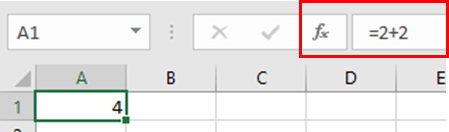Every function must start with equals to sign (=). It tells the excel that the user needs to access Functions in the sheet. Now see the same operation between two values using cell reference.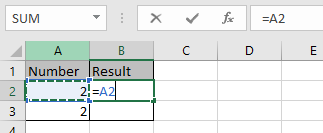Whenever you will using cell reference. You just need to use the equals to(=) sign and just click the cell where your values are.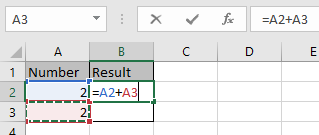Excel helps you to differentiate between the 2 cell references with the different colour format as shown in the above snapshot. Now press Enter and you will have your result.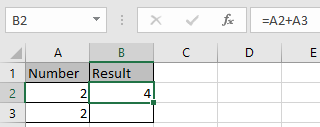Now you can use this output into the input of another function. If the values are text, then we need to take it as strings and apply the functions appropriately.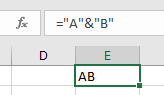Here string “A” is joined with string “B” using & operator to get the new text “AB” in the cell.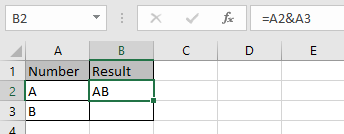Now there’s one more thing. Numbers represent so many values in a text as Date, Time and currency. So Sometimes you need to change the format cell type to required field type using Format cell option.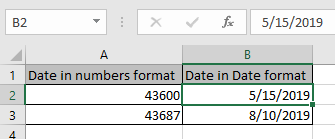Use the format cell option shown below or Ctrl + 1 shortcut keyboard key that will open a full Format cell dialog box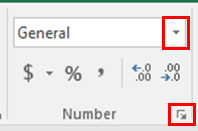Changing the format you will get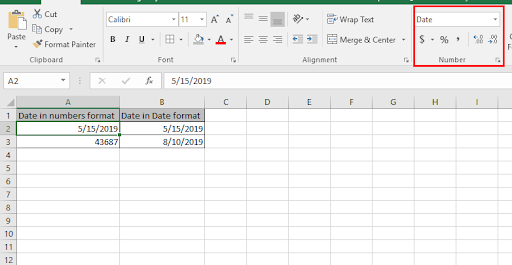Here are all Excel Functions with their Description and Syntax.

### How to use the DATE function in Excel

The Excel DATE function is a DATE & TIME function. This function returns the DATE value in numerical format. Convert the output to date format...

### How to use the LINEST function in Excel

In this article, we will learn How to use the LINEST function in Excel. Equation of line: If there is a single range of x-values, the calc...

### How to concatenate or merge in Excel

In this article, we will learn How to concatenate or merge in Excel. What is Concatenate in Excel? In General, there are two ways to combine d...

### How to Get net working days in Excel

In this article, we will learn How to Get net working days in Excel. What are working and non working days ? Usually Saturdays and Sundays a...

### How to use the PMT function in Excel

In this article, we will learn How to use the PMT function in Excel. What is this PMT function used to calculate a loan ? Excel let’s a pe...

### How to use the Z.TEST function in Excel

In this article, we will learn How to use the Z.TEST function in Excel. What is hypothesis testing and how to use Z -Test for  hypothesis test...

### How to use the T.INV function in Excel

In this article, we will learn How to use the T.INV function in Excel. What is hypothesis testing, Inverse of T distribution and degree of free...

Terms and Conditions of use

The applications/code on this site are distributed as is and without warranties or liability. In no event shall the owner of the copyrights, or the authors of the applications/code be liable for any loss of profit, any problems or any damage resulting from the use or evaluation of the applications/code.